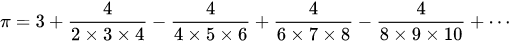Share your repls and programming experiences

← Back to all posts

# Pi Calculator

So, I wanted to make a Pi calculator without imports. I figured I could just use circles, but I would have to use Pi to render the circles in Python without imports (to my knowledge)... So, in search of calculating Pi, I came upon the Leibniz formula. My script started out about 100 lines long but I got it down real small; however, though, this formula was very inefficient, and I ended up with the Nilakantha formula. It's really fast and generates a lot of digits. I'm kinda proud of it, so I hope you guys like it too. If anyone knows how I could make it faster or more accurate, I'm open to any suggestions!

##### Comments
hotnewtop
DynamicSquid (4622)

Nice! How does it work?

DuckMonkey (6)

@DynamicSquid glad you asked! let me break it down:

# The range

The range is set to count up to n plus one (the number that the user inputs) from two, by four...

For example, if the user were to put in 10, the range would count up like so:

`2, 6, 10`

# The formula

The formula used in this one is the Nilakantha sequence, or in this, 4/(i*(i+1)*(i+2)) - 4/((i+2)*(i+3)*(i+4)).

Here's what the formula looks like:# Also

Sadly, none of the formulas I've tried exceed 14 digits, and not just because a decimal in a string is that long by default.

Even if I were to extend the digits with a module or using this:

`'%.100f' % n`

...the digits aren't accurate after 14, no matter how many iterations you push into it [, sadly].

I don't know what the problem is with it but I'm aiming to fix it :/

DynamicSquid (4622)

@DuckMonkey Ah okay, cool! Thanks for sharing :)

DuckMonkey (6)

@DynamicSquid Of course, thanks for the upvote :)